#### FM442: Quantitative Methods for Finance and Risk Analysis

Jon Danielsson
Michaelmas Term 2020

# Seminar 3

In this class we will:

We first load the packages for this seminar:

In :
library(tseries)
library(car)
library(lubridate)


## Statistical distributions¶

We need to be comfortable working with Probability Distributions in order to build financial risk forecasting models. In particular, we are going to work with the Normal Distribution, the Student-T, and the Chi-Square distribution. The names of these distributions in R, respectively, are: norm, t, and chisq.

Every distribution in R has four functions:

• p for "probability, or the cumulative distribution function (cdf)
• q for "quantile", the inverse of the cdf
• d for density, the probability density function (pdf)
• r for "random", a random variable drawn from the specified distribution

To apply any of these, you just need to add them before the distribution's name.

For example, we can use pnorm to calculate the cdf: $$F(x) = P(X≤x)$$ Where $X$ is a normally distributed random variable.

In :
# CDF of 0 under a Standard Normal
pnorm(0)

0.5
In :
# CDF of 8 under a Normal with mean 10 and standard deviation 2
pnorm(8, mean = 10, sd = 2)

# Rounding it
round(pnorm(8, mean = 10, sd = 2),3)

0.158655253931457
0.159
In :
# Sequence from -2 to 2, with increments of 0.5
sequence <- seq(-2, 2, 0.5)
round(pnorm(sequence),3)

1. 0.023
2. 0.067
3. 0.159
4. 0.309
5. 0.5
6. 0.691
7. 0.841
8. 0.933
9. 0.977

For many applications, we will be interested in looking for the inverse of the cdf. For example, if we want to find the 95-th quantile of a Student-t with 3 degrees of freedom:

In :
# Inverse CDF of 0.95 under a Student-t with 3 degrees of freedom
qt(0.95, df = 3)

2.35336343480182

### Plotting a distribution¶

We can read probability-quantile combinations from the CDF plot of a distribution. Let's find the 5% quantile of a Standard Normal:

In :
# Creating a sequence
x <- seq(-3,3,0.1)

# Vector with the CDF
cdf <- pnorm(x)

# Plotting it
plot(x, cdf, type = "l", main = "CDF of a Standard Normal",
lwd = 3, las = 1, col = "blue")

# Find 5% quantile using qnorm
q5 <- qnorm(0.05)

# Add lines using the segments() function
segments(x0 = q5, y0 = -1, y1 = 0.05, lty = "dashed", lwd = 2, col ="red")
segments(x0 = -4, y0 = 0.05, x1 = q5, lty = "dashed", lwd = 2, col ="red")

# Add tick marks in the plot
axis(1, at = q5, label = round(q5,2))
axis(2, at = 0.05, label = 0.05, las = 1)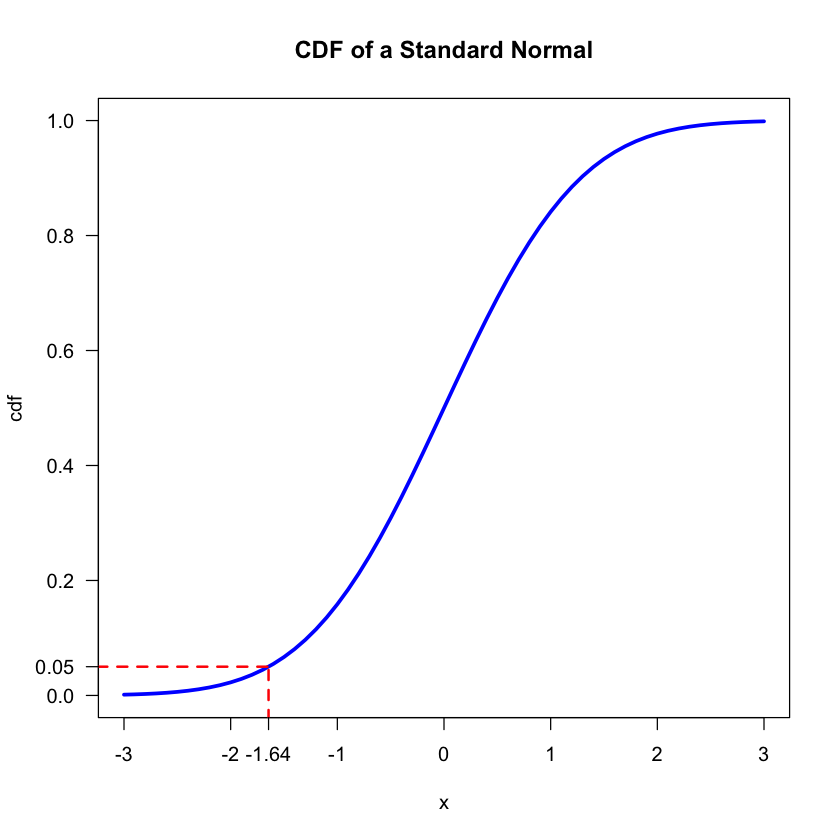### Comparing the Normal Distribution with the Student-t¶

The Student-t distribution has fatter tails than the Normal, which can be convenient for some applications. The lower the degrees of freedom of a Student-t, the fatter the tails. By definition, a Student-t will converge to a Normal distribution as the degrees of freedom go to infinity.

In :
# Start by creating a sequence
x <- seq(-3, 3, 0.1)

# Normal density
normal <- dnorm(x)

# Student-t with 2 df
st2 <- dt(x, df = 2)

# Student-t with 3 df
st3 <- dt(x, df = 3)

# Student-t with 10 df
st10 <- dt(x, df = 10)

plot(x, normal, type = "l", main = "Comparing distributions", col = 1, xlab = "x", ylab = "f(x)")
lines(x, st2, col = 2)
lines(x, st3, col = 3)
lines(x, st10, col = 4)

legend("topright", legend = c("Normal", "T - 2 df", "T - 3 df", "T - 10 df"), col = c(1:4), fill = c(1:4))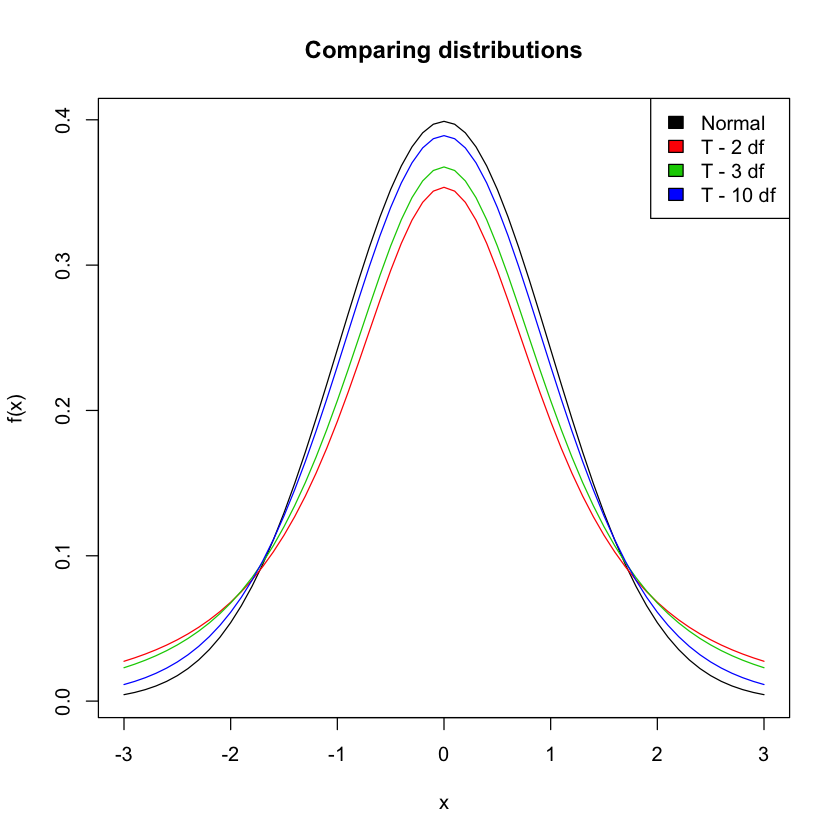## Random numbers and Monte Carlo¶

One of the advantages of working with statistical software is being able to do simulations using random (or better said, pseudorandom) numbers. We will go into more details on random numbers generation in Chapter 7.

We can get random numbers drawn from a specific distribution by using the prefix r before the distribution name:

In :
# One random number from a Standard Normal
rnorm(1)

# Ten random numbers from a Normal(10, 0.5)
rnorm(10, mean = 10, sd = 0.5)

# One random number from a Student-t with 5 degrees of freedom
rt(1, df = 5)

0.937012408830822
1. 9.27746310496962
2. 10.031635897445
3. 10.1746320467716
4. 10.7681963935134
5. 9.74040713059832
6. 9.92440986730903
7. 8.75117935351729
8. 9.76837828386729
9. 10.8255383105505
10. 9.58157505138012
-0.31485745875495

Everytime we run a function that outputs random numbers, we will get a different value. Usually, to allow for replication, we set a seed:

In :
set.seed(442)
rnorm(5)

1. -0.894891360378309
2. -2.6921679536767
3. 0.764099073417597
4. -0.0172356169957856
5. -1.62406009659155
In :
set.seed(442)
rnorm(5)

1. -0.894891360378309
2. -2.6921679536767
3. 0.764099073417597
4. -0.0172356169957856
5. -1.62406009659155

### Comparing distributions using random numbers¶

To show the Fat Tails of the Student-t compared to the Normal distribution, we will draw 1000 points from each distribution:

In :
# Normal distribution
rnd_normal <- rnorm(1000)

# Student-t
rnd_t <- rt(1000, df = 3)

# Plotting
plot(rnd_t, col = "red", pch = 16, main = "Random points from a Normal and Student-t")
points(rnd_normal, col = "blue",pch = 16)

legend("bottomright", legend = c("Student-t", "Normal"), pch = 16, col = c("red", "blue"))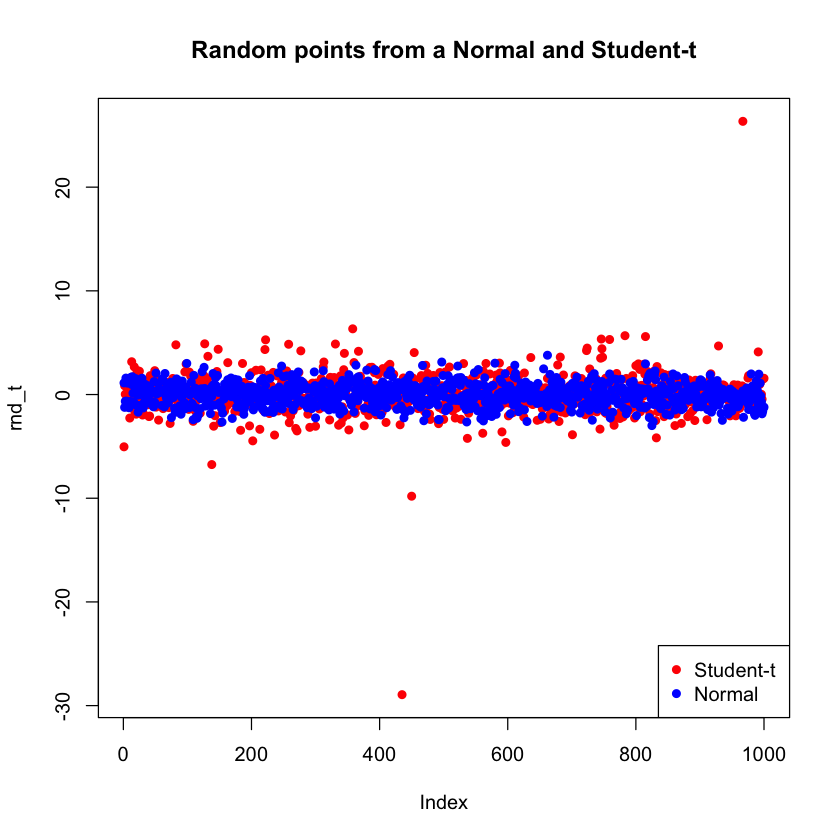We see that the points from a Student-t distribution take on more extreme values compared to the Normal. This is a consequence of fat tails.

Let's say I want to assign your grades for FM442 using a random number generator. Grades go from 0 to 100 and have to be integers. Drawing numbers from a distribution like the Normal or the Uniform will give us non-integers, so we can either:

• Round the number to the nearest integer
• Use a different function, like sample(), which only outputs integers

We will use the latter. sample(x,size) takes a vector x, and a size, and returns a vector of the given size of random draws from x:

In :
sample(1:100, 1)
sample(1:100, 3)
sample(1:100, 5)

44
1. 53
2. 10
3. 37
1. 9
2. 31
3. 20
4. 18
5. 86

Let's say there are 60 students in the class, we can get the grades:

In :
# Getting the grades

# Creating a histogram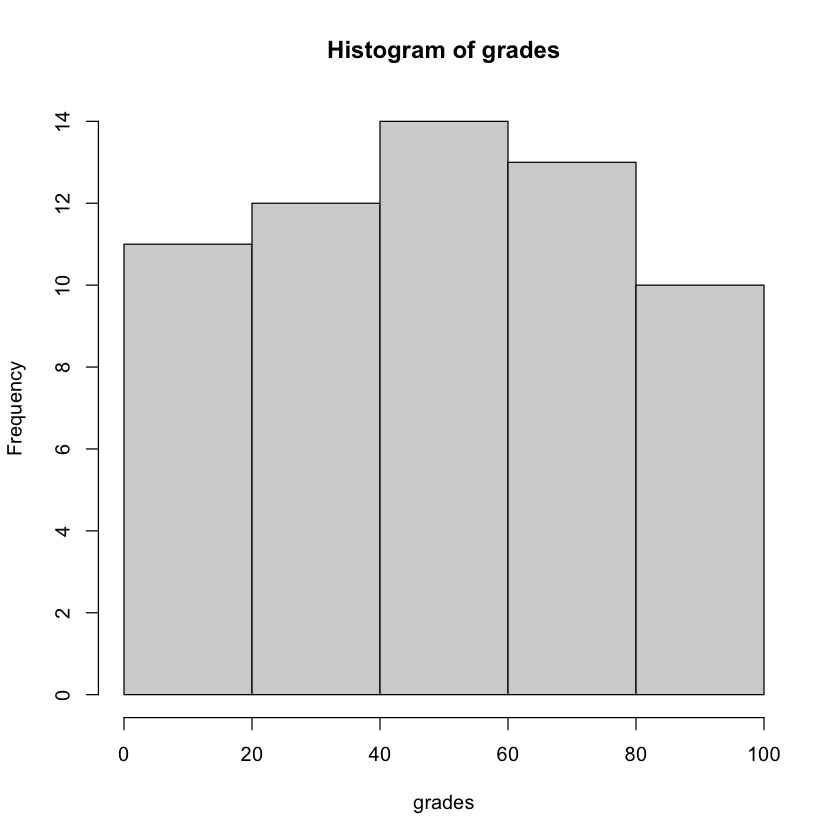How many people got a Distinction:

In :
# We can use a condition in the square brackets to subset the vector:

18

# Small and Large sample properties¶

Let's explore the distribution of random samples of different sizes, drawn from a standard normal distribution, compared to the distribution:

In :
# Sample size: 100
norm1 <- rnorm(100)

# Sample size: 1000
norm2 <- rnorm(1000)

# Sample size: 100000
norm3 <- rnorm(100000)

# Create a sequence
x <- seq(-3,3,0.1)

# Plot the histograms and overlay a normal distribution
hist(norm1, freq = FALSE, breaks = 20, main = "Sample size 100", col = "lightgrey", ylim = c(0, 0.5))
lines(x,dnorm(x), lwd = 3, col = "red")
hist(norm2, freq = FALSE, breaks = 20, main = "Sample size 1000", col = "lightgrey", ylim = c(0, 0.5))
lines(x,dnorm(x), lwd = 3, col = "red")
hist(norm3, freq = FALSE, breaks = 20, main = "Sample size 100000", col = "lightgrey", ylim = c(0, 0.5))
lines(x,dnorm(x), lwd = 3, col = "red")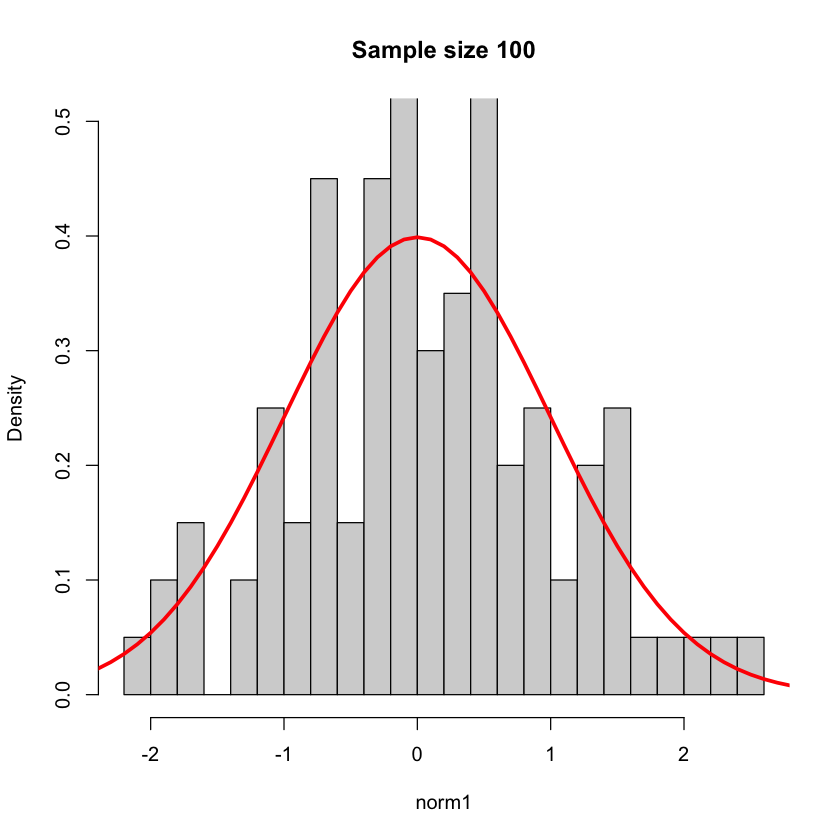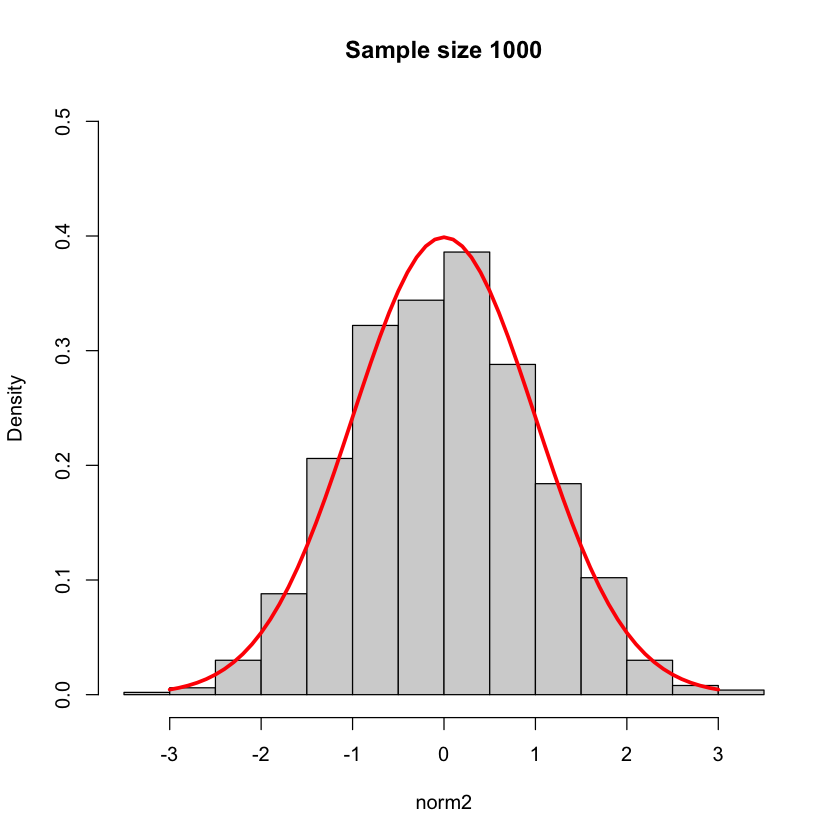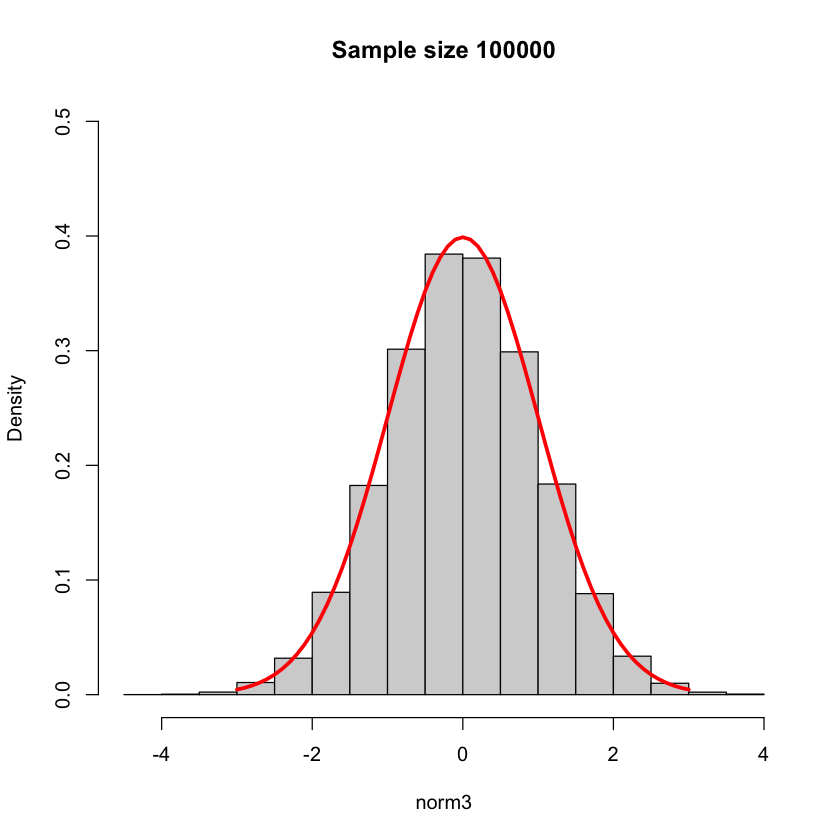### Monte Carlo¶

A Monte Carlo method is a class of computational algorithm that uses repeated random sampling to obtain numerical results. It is commonly used in quantitative finance to simulate uncertainty and analyze instruments, portfolios or investments.

We are going to do a simple exercise to simulate the profit and loss distribution from holding a stock.

Assume we have a stock that has normally distributed returns with mean $0$ and variance $\sigma^{2}$, and a risk-free interest rate $r$. We can then simulate $S$ one-day returns:

$$y_{t+1,i} \sim \mathcal{N}\left(0,\sigma^{2}\right), i = 1, ..., S$$

And with each simulated return, calculate the one-day future price following the formula. The last term is the lognormal correction. We will discuss this in detail in chapter 7:

$$P_{t+1,i} = P_{t}e^{r(1/365)} \times e^{y_{t+1,i}} \times e^{-0.5\sigma^{2}}$$

Consider a stock $X$ with price $100$, and daily volatility $0.01$. The annual risk free rate is $5\%$. We will find the distribution of profits and loss for tomorrow's price.

In :
# Initial price
X_0 <- 100

# Volatility
sigma <- 0.01

# Interest rate
r <- 0.05

# Initialize a vector that will contain all realizations
X_1 <- numeric()

# Perform one million realizations
for (i in 1:10^6) {
X_1[i] <- X_0*exp(r/365)*exp(rnorm(1,0,sigma)-sigma^2/2)
}

# Plot a histogram
hist(X_1, col = "lightgray", main = "Distribution of expected prices",
xlab = "Price")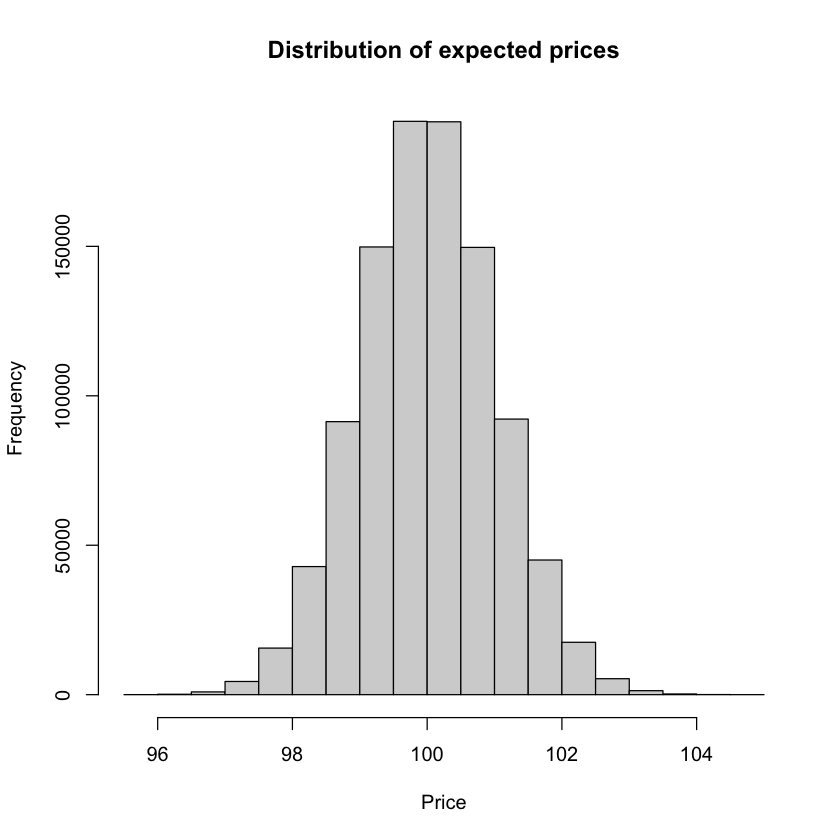We don't know what tomorrow's price will be, but based on the assumptions we made, this experiment gives us the possible values.

We can order the values and find the quantile of this distribution using the quantile() function.

Let's find what is the 5% quantile of our expected price:

In :
quantile(X_1, 0.05)

5%: 98.3791034901985

Adding the 5% quantile as a vertical line in the plot:

In :
hist(X_1, col = "lightgray", main = "Distribution of expected prices",
xlab = "Price")

abline(v = quantile(X_1, 0.05), lwd = 2, col = "red")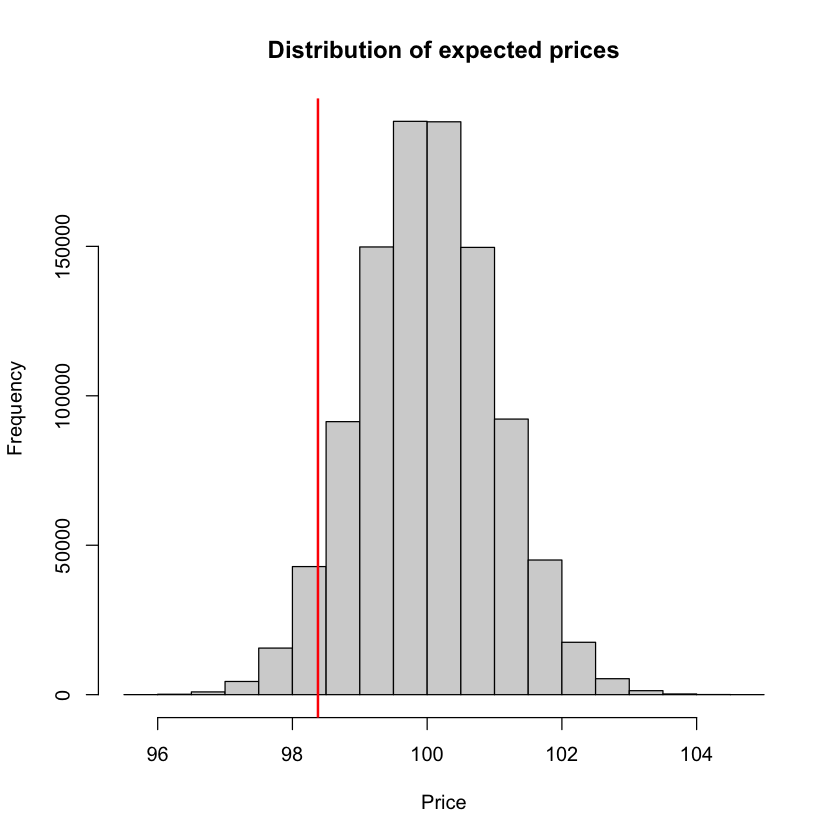### Random Walk¶

A random walk is a stochastic process that describe the path of a variable that moves randomly through time. For example, consider the process:

$$Y_{t} = Y_{t-1} + \epsilon_{t}$$

Where $Y_{0}$ is given and $\epsilon \sim \mathcal{N}\left(0,\sigma^2\right)$.

We can consider a stock, $Y$, that at time $0$ has price of 500, and follows a random walk with $\sigma^2 = 2$ . If we want to see how the price evolves over time, we can use R to create a large number of simulations. Let's see one thousand different simulations on how the price of the stock would move in one hundred days:

In :
# Create a matrix to hold the paths. One column for every simulation and one row for each day, starting from 0
mtx <- matrix(NA, nrow = 101, ncol = 1000)

# Since at time 0 the price is 500, we fill the first row with this value
mtx[1,] <- 500

# Use a loop to draw the path of the price for each simulation

# i represents the simulation, from 1 to 1000
for (i in 1:1000) {

# j represents the row in our matrix, one for each day
for (j in 2:101) {

# We proceed to fill the matrix following the random walk
mtx[j,i] <- mtx[j-1,i] + rnorm(1, mean = 0, sd = 2.5)
}
}


We have run 1000 simulations, each starts with a price of 100 and follows a random walk for 100 time periods. Let's see the head of the matrix:

In :
head(mtx)

 500 500 500 500 500 500 500 500 500 500 ⋯ 500 500 500 500 500 500 500 500 500 500 497.473 500.614 500.709 501.24 498.207 502.236 499.594 504.631 500.866 499.251 ⋯ 497.063 501.601 497.075 502.659 501.05 495.356 502.541 500.01 501.836 500.909 500.494 503.946 501.256 502.506 505.073 502.308 501.088 501.927 503.671 497.72 ⋯ 500.822 500.395 496.369 502.471 505.925 498.371 504.21 500.527 504.822 500.305 496.345 503.362 502.196 501.887 506.176 501.292 498.669 496.95 498.951 493.367 ⋯ 500.217 500.588 493.67 495.295 503.885 498.822 504.363 497.824 508.204 497.83 500.131 505.973 504.092 499.489 505.758 504.504 498.298 498.358 495.151 496.651 ⋯ 505.403 498.579 490.444 495.377 502.227 501 503.499 503.489 507.281 494.124 496.688 507.561 505.279 508.414 509.634 500.833 497.881 498.567 495.433 500.379 ⋯ 508.802 496.985 492.267 495.215 495.765 488.369 501.954 501.748 505.973 493.209

We can plot it using matplot() to see the random walk visually:

In :
matplot(mtx, main = "Random Walk", xlab = "Time", ylab = "Price", type = "l", lty = 1)